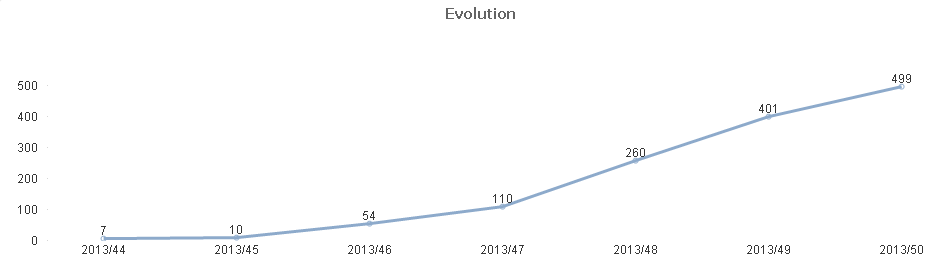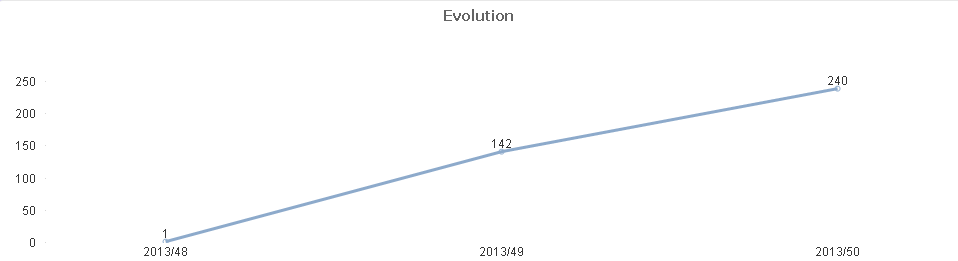QlikView Creating Analytics

Discussion Board for collaboration related to Creating Analytics for QlikView.

HighlightedNot applicable

Full accumulation after filter

How can I still have the full accumulation in a chart, after select a filter?

When I filter, I want the first value to be accumulated with the all the previous values ones. (althoguh they are not selected).

For example:

I have this chart:When I select November, the chart is:And if I select December I have this one:In december I want the full accumulation, not just the data from december. So, instead of 1, 143, 240, I want the 260, 401, 499.

I've tried with total, all...

Tags (4)
1 Solution

Accepted Solutions
Contributor III

Re: Full accumulation after filter

Sorry,

in my graph I used expression

rangesum(above(total sum({\$<Date= >}Sales), 0, rowno(Total)))  + Sum(TOTAL {<Month={"\$(=min(Month)-1)"}>} Sales)

rangesum(above(total sum({\$<Date= >}Sales), 0, rowno(Total))) this is regular sum above for a chosen month  month

+ Sum(TOTAL {<Month={"\$(=min(Month)-1)"}>} Sales) this add value from previous month - you need to have month in calendar.

Best Regards,

Anna

9 RepliesNot applicable

Re: Full accumulation after filter

I've also tried:

sum( {<Date>} , Id, 0))

I have the correct values, but the chart show all the weeks and not only December.

Contributor III

Contributor III

Re: Full accumulation after filter

Hi Jose,

How do you set week/year? You need set expression to get values from the first day of the week no matter if month is A or B.

-JFlorian

Contributor III

Re: Full accumulation after filter

I just find out that my previous answer  it is not what you are looking forSee new fileNot applicable

Re: Full accumulation after filter

I cant open your document, because I can't access with License Qlikview, and I am using a Personal Edition.Not applicable

Re: Full accumulation after filter

What do you mean by setting the expression?

I have a table with a date. That day, is link to another table (calendar table), from where I choose the field to select WeekName (Date), in this case. So Week 48, is in November and December.

I am basically using this expression: sum (idOrder), (idOrder is always 1, so i'm counting rows) with Full Accumulation.

Contributor III

Re: Full accumulation after filter

I think is not the best way but...

I work with expression, where we call min date in the month selected "Min(Fecha)"... so, we calculated the weekstart and then week to the year...

=Sum(

{<Semana_Año={">=\$(=Week(WeekStart(Min(Fecha))))<=\$(=Week(WeekStart(Min(Fecha)))+4)"}>}

idOrder)

I hope you can understand me...

-JFlorian

Contributor III

Re: Full accumulation after filter

Sorry,

in my graph I used expression

rangesum(above(total sum({\$<Date= >}Sales), 0, rowno(Total)))  + Sum(TOTAL {<Month={"\$(=min(Month)-1)"}>} Sales)

rangesum(above(total sum({\$<Date= >}Sales), 0, rowno(Total))) this is regular sum above for a chosen month  month

+ Sum(TOTAL {<Month={"\$(=min(Month)-1)"}>} Sales) this add value from previous month - you need to have month in calendar.

Best Regards,

AnnaNot applicable

Re: Full accumulation after filter

Wow!!

Thank you so much! That worked perfectly.

Kind regards.